Ex 10.2

Chapter 10 Class 11 Straight Lines (Term 1)
Serial order wise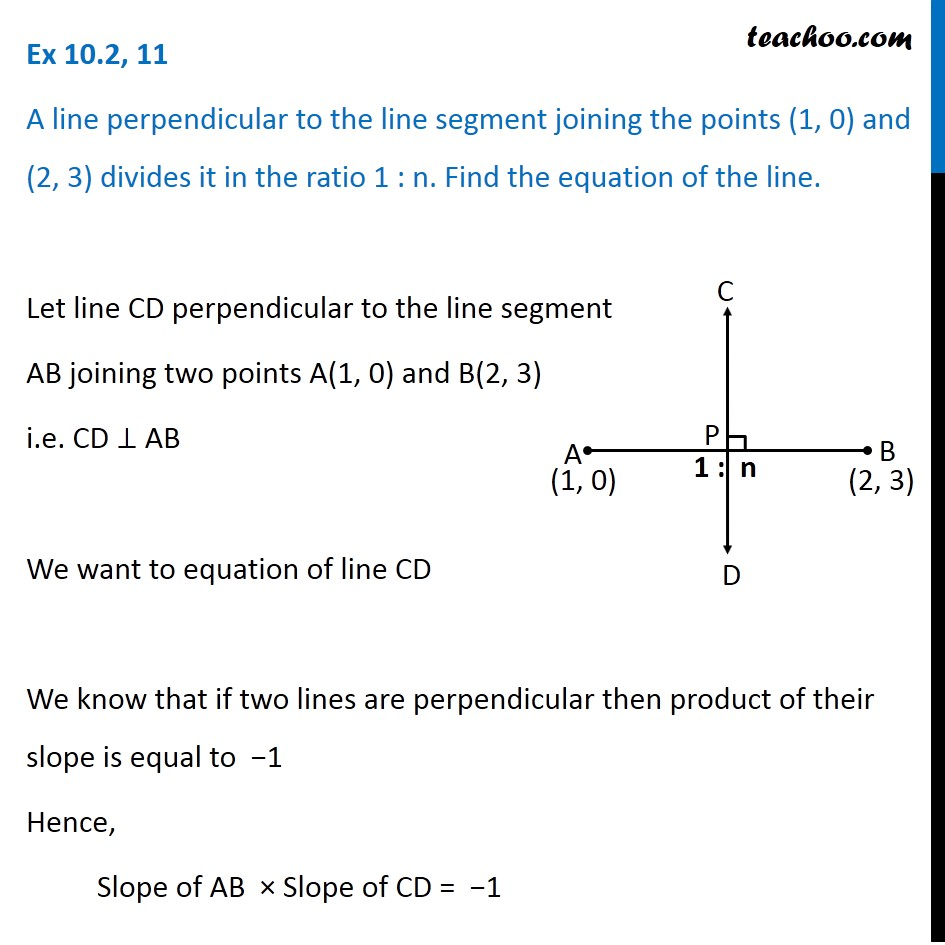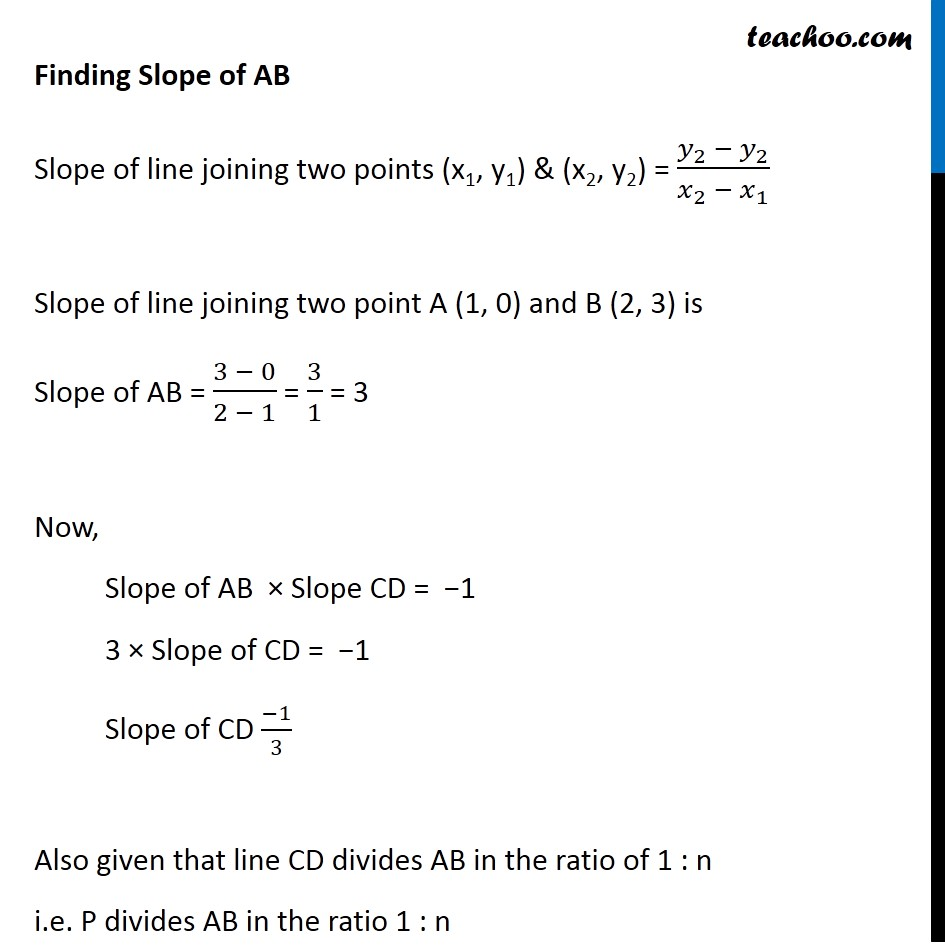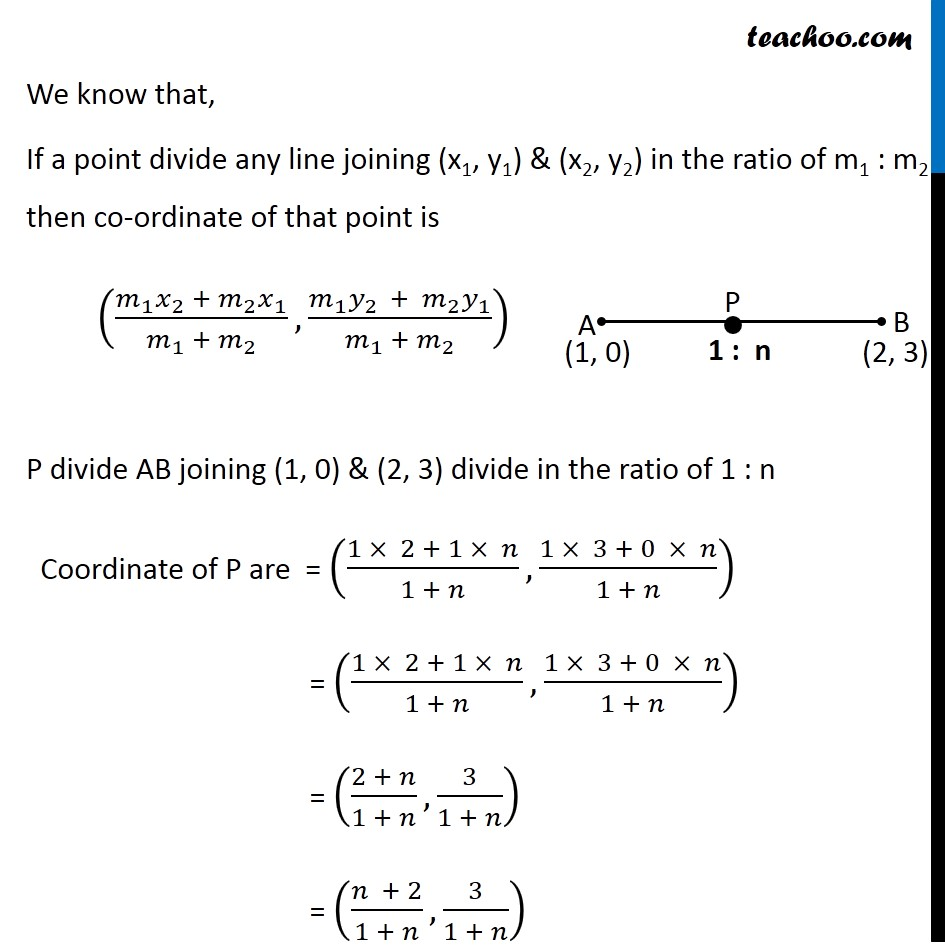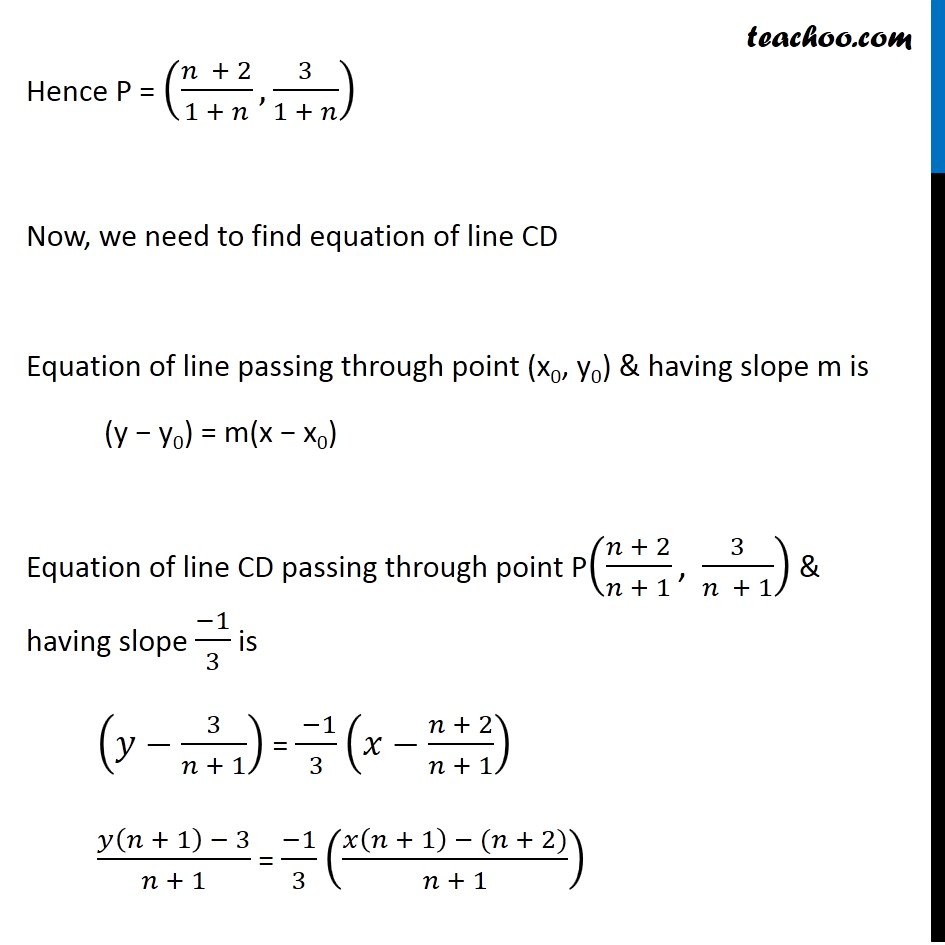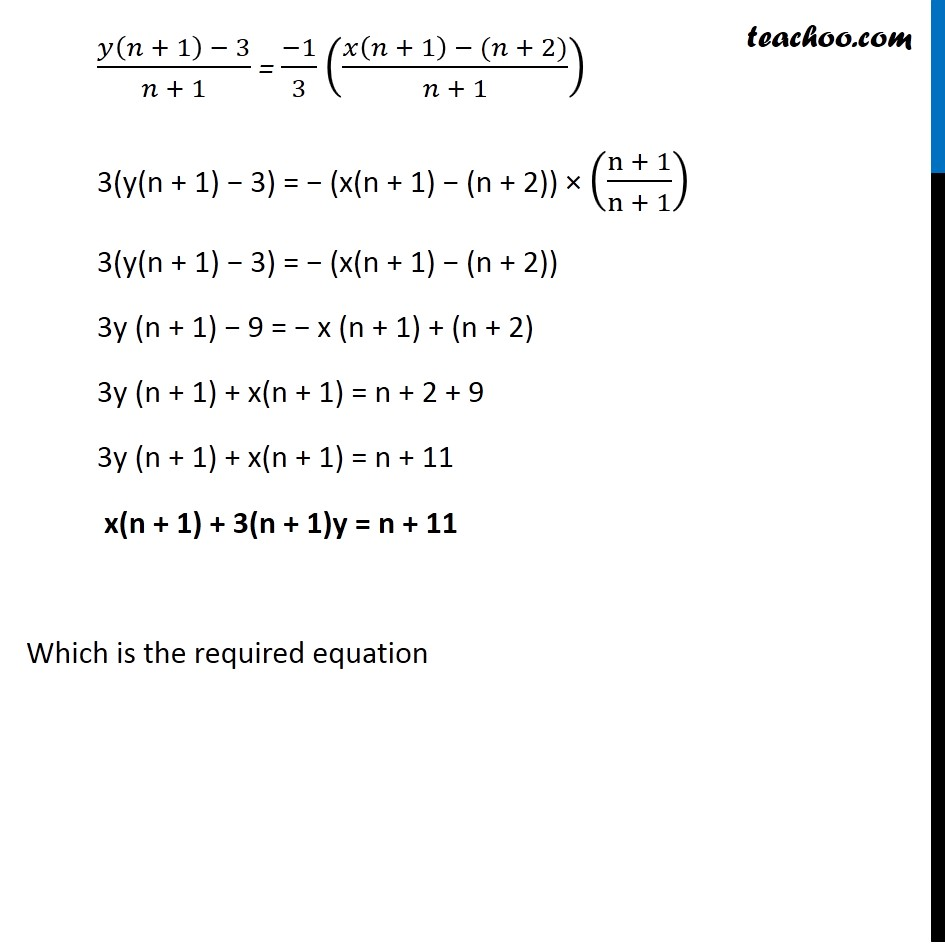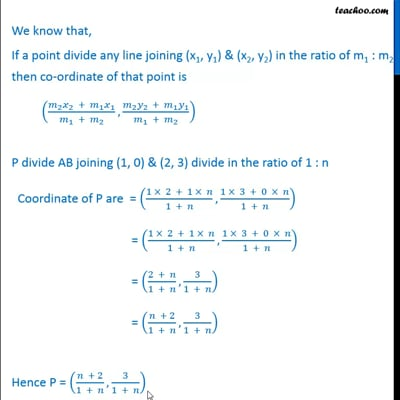This video is only available for Teachoo black users

### Transcript

Ex 10.2, 11 A line perpendicular to the line segment joining the points (1, 0) and (2, 3) divides it in the ratio 1 : n. Find the equation of the line. Let line CD perpendicular to the line segment AB joining two points A(1, 0) and B(2, 3) i.e. CD ⊥ AB We want to equation of line CD We know that if two lines are perpendicular then product of their slope is equal to −1 Hence, Slope of AB × Slope of CD = −1 Finding Slope of AB Slope of line joining two points (x1, y1) & (x2, y2) = (𝑦_2 − 𝑦_2)/(𝑥_2 − 𝑥_1 ) Slope of line joining two point A (1, 0) and B (2, 3) is Slope of AB = (3 − 0)/(2 − 1) = 3/1 = 3 Now, Slope of AB × Slope CD = −1 3 × Slope of CD = −1 Slope of CD (−1)/3 Also given that line CD divides AB in the ratio of 1 : n i.e. P divides AB in the ratio 1 : n We know that, If a point divide any line joining (x1, y1) & (x2, y2) in the ratio of m1 : m2 then co-ordinate of that point is ((𝑚_1 𝑥_2 + 〖𝑚_2 𝑥〗_1)/(𝑚_1 + 𝑚_2 ),(𝑚_1 𝑦_(2 ) + 𝑚_2 𝑦_1)/(𝑚_1 + 𝑚_2 )) P divide AB joining (1, 0) & (2, 3) divide in the ratio of 1 : n Coordinate of P are = ((1 × 2 + 1 × 𝑛)/(1 + 𝑛), (1 × 3 + 0 × 𝑛)/(1 + 𝑛)) = ((1 × 2 + 1 × 𝑛)/(1 + 𝑛), (1 × 3 + 0 × 𝑛)/(1 + 𝑛)) = ((2 + 𝑛)/(1 + 𝑛), 3/(1 + 𝑛)) = ((𝑛 + 2)/(1 + 𝑛), 3/(1 + 𝑛)) Hence P = ((𝑛 + 2)/(1 + 𝑛), 3/(1 + 𝑛)) Now, we need to find equation of line CD Equation of line passing through point (x0, y0) & having slope m is (y − y0) = m(x − x0) Equation of line CD passing through point P((𝑛 + 2)/(𝑛 + 1) ", " 3/(𝑛 + 1)) & having slope (−1)/3 is (𝑦−3/(𝑛 + 1)) = ( −1)/3 (𝑥−(𝑛 + 2)/(𝑛 + 1)) (𝑦(𝑛 + 1) − 3)/(𝑛 + 1) = (−1)/3 ((𝑥(𝑛 + 1) − (𝑛 + 2))/(𝑛 + 1)) (𝑦(𝑛 + 1) − 3)/(𝑛 + 1) = (−1)/3 ((𝑥(𝑛 + 1) − (𝑛 + 2))/(𝑛 + 1)) 3(y(n + 1) − 3) = − (x(n + 1) − (n + 2)) × ((n + 1)/(n + 1)) 3(y(n + 1) − 3) = − (x(n + 1) − (n + 2)) 3y (n + 1) − 9 = − x (n + 1) + (n + 2) 3y (n + 1) + x(n + 1) = n + 2 + 9 3y (n + 1) + x(n + 1) = n + 11 x(n + 1) + 3(n + 1)y = n + 11 Which is the required equation